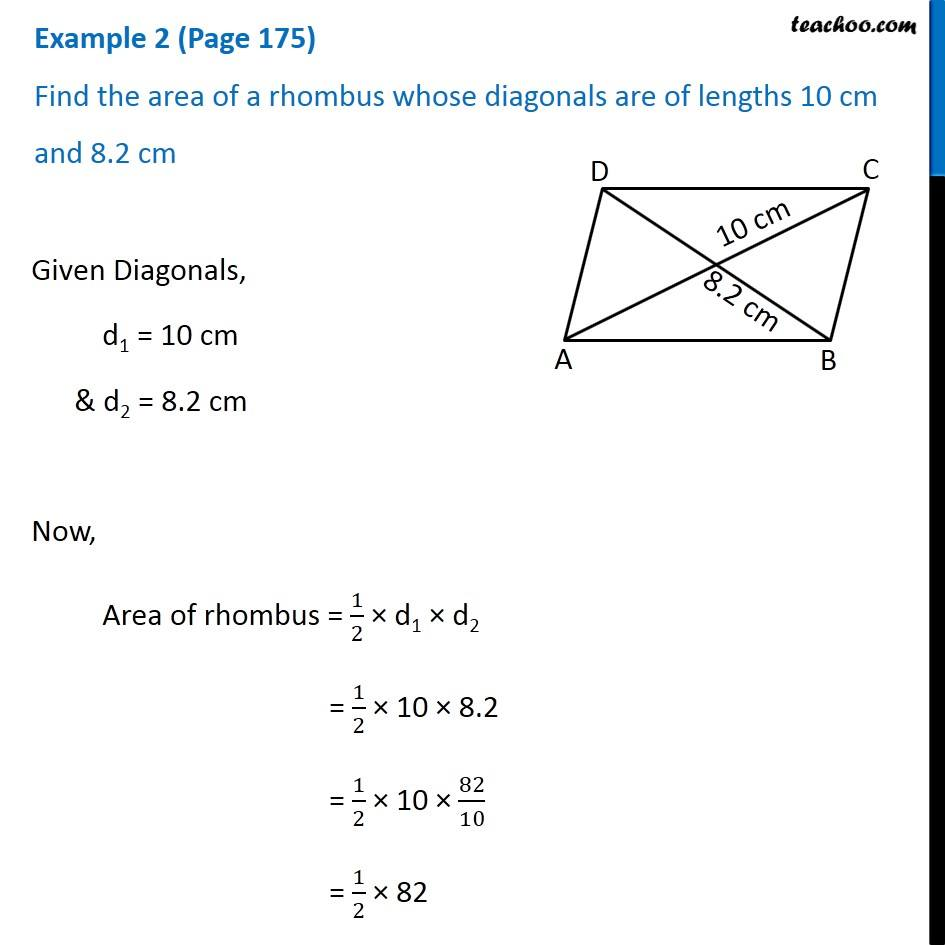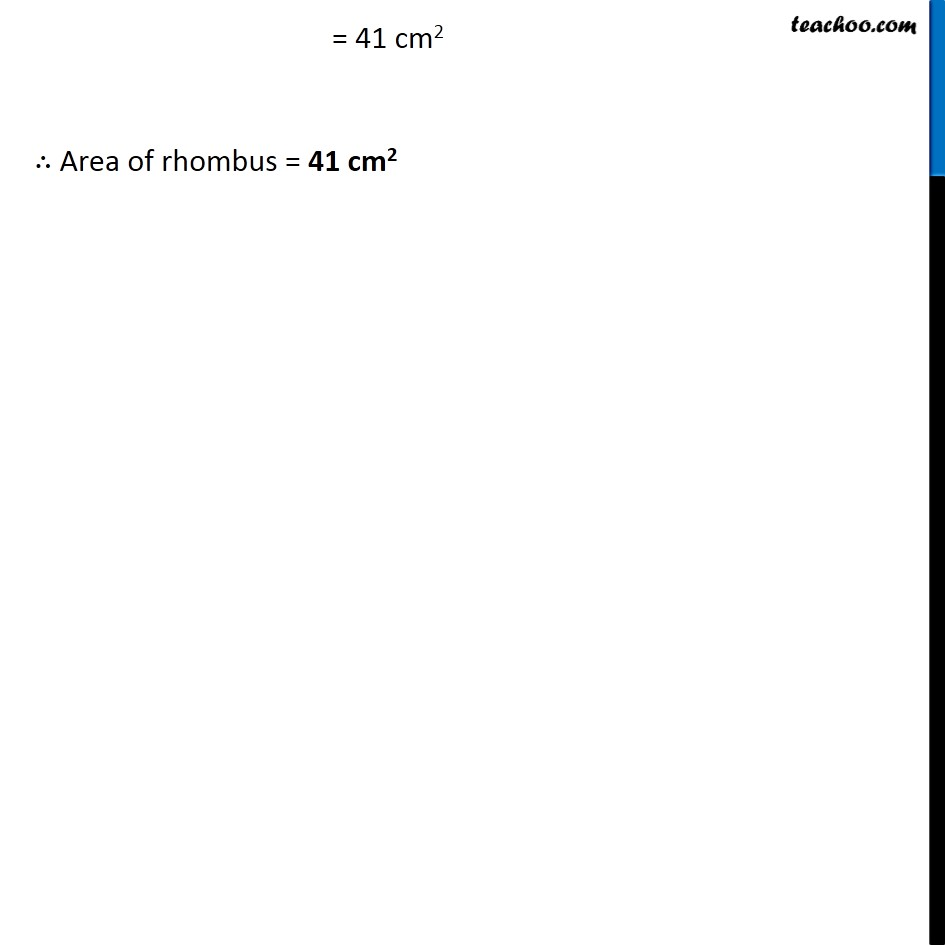Examples

Chapter 9 Class 8 Mensuration
Serial order wiseLearn in your speed, with individual attention - Teachoo Maths 1-on-1 Class

### Transcript

Question 1 (Page 175) Find the area of a rhombus whose diagonals are of lengths 10 cm and 8.2 cm Given Diagonals, d1 = 10 cm & d2 = 8.2 cm Now, Area of rhombus = 1/2 × d1 × d2 = 1/2 × 10 × 8.2 = 1/2 × 10 × 82/10 = 1/2 × 82 = 41 cm2 ∴ Area of rhombus = 41 cm2